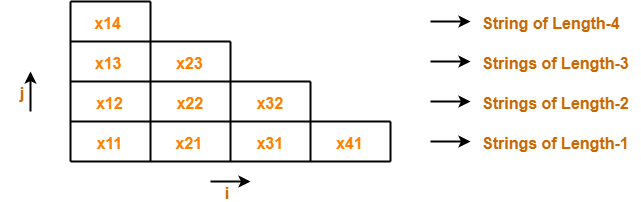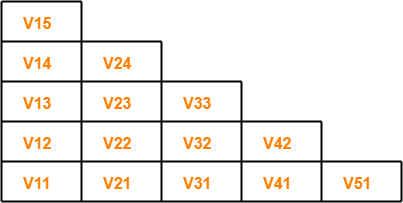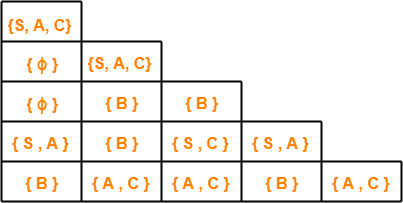# CYK Algorithm | CYK Algorithm Example

## CYK Algorithm-

 CYK Algorithm is a membership algorithm of context free grammar.

• It is used to decide whether a given string belongs to the language of grammar or not.
• It is also known as CKY Algorithm or Cocke-Younger-Kasami Algorithm after its inventors.

## Important Notes-

### Note-02:

• The worst case running time of CYK Algorithm is Θ (n3.|G|).
• Here, n = Length of parsed string and |G| = Size of the CNF Grammar G.

## Algorithm-

```Begin
for ( i = 1 to n do )
Vi1 { A | A → a is a production where ith symbol of x is a }

for ( j = 2 to n do )
for ( i = 1 to n - j + 1 do )
Begin
Vij = ϕ
For k = 1 to j - 1 do
Vij = Vij ∪ { A | A → BC is a production where B is in Vik and C is in V(i + k)(j - k) }
End
End```

Also Read- Algorithm To Check Whether CFL is Empty

## Deciding Membership Using CYK Algorithm-

To decide the membership of any given string, we construct a triangular table where-

• Each row of the table corresponds to one particular length of sub strings.
• Bottom most row corresponds to strings of length-1.
• Second row from bottom corresponds to strings of length-2.
• Third row from bottom corresponds to strings of length-3.
• Top most row from bottom corresponds to the given string of length-n.

## Example-

For a given string “x” of length 4 units, triangular table looks like-We will fill each box of xij with its Vij.

These notations are discussed below.

## Notations Used

### Notation-01: xij

xij represents a sub string of “x” starting from location ‘i’ and has length ‘j’.

Example-

Consider x = abcd is a string, then-

Number of sub strings possible = n(n+1)/2 = 4 x (4+1) / 2 = 10

We have-

• x11 = a
• x21 = b
• x31 = c
• x41 = d
• x12 = ab
• x22 = bc
• x32 = cd
• x13 = abc
• x23 = bcd
• x14 = abcd

### Notation-02: Vij

Vij represents a set of variables in the grammar which can derive the sub string xij.

If the set of variables consists of the start symbol, then it becomes sure-

• Sub string xij can be derived from the given grammar.
• Sub string xij is a member of the language of the given grammar.

Also Read- Algorithm To Check Whether CFL is Finite

## Problem-

For the given grammar, check the acceptance of string w = baaba using CYK Algorithm-

S → AB / BC

A → BA / a

B → CC / b

C → AB / a

## Solution-

First, let us draw the triangular table.

• The given string is x = baaba.
• Length of given string = |x| = 5.
• So, Number of sub strings possible = (5 x 6) / 2 = 15.

So, triangular table looks like-Now, let us find the value of Vij for each cell.

## For Row-1:

### Finding V11

• V11 represents the set of variables deriving x11.
• x11 = b.
• Only variable B derives string “b” in the given grammar.
• Thus, V11 = { B }

### Finding V21

• V21 represents the set of variables deriving x21.
• x21 = a.
• Variables A and C derive string “a” in the given grammar.
• Thus, V21 = { A , C }

### Finding V31

• V31 represents the set of variables deriving x31.
• x31 = a.
• Variables A and C derive string “b” in the given grammar.
• Thus, V31 = { A , C }

### Finding V41

• V41 represents the set of variables deriving x41.
• x41 = b.
• Only variable B derives string “b” in the given grammar.
• Thus, V41 = { B }

### Finding V51

• V51 represents the set of variables deriving x51.
• x51 = a.
• Variables A and C derives string “a” in the given grammar.
• Thus, V51 = { A , C }

## For Row-2-

### RULE

As per the algorithm, to find the value of Vij from 2nd row on wards,

we use the formula-

Vij = Vik V(i+k)(j-k)

where k varies from 1 to j-1

### Finding V12

We have i = 1 , j = 2 , k = 1

Substituting values in the formula, we get-

V12 = V11. V21

V12 = { B } { A , C }

V12 = { BA , BC }

∴ V12 = { A , S }

### Finding V22

We have i = 2 , j = 2 , k = 1

Substituting values in the formula, we get-

V22 = V21. V31

V22 = { A , C } { A , C }

V22 = { AA , AC , CA , CC }

Since AA , AC and CA do not exist, so we have-

V22 = { CC }

∴ V22 = { B }

### Finding V32

We have i = 3 , j = 2 , k = 1

Substituting values in the formula, we get-

V32 = V31. V41

V32 = { A , C } { B }

V32 = { AB , CB }

Since CB does not exist, so we have-

V32 = { AB }

∴ V32 = { S , C }

### Finding V42

We have i = 4 , j = 2 , k = 1

Substituting values in the formula, we get-

V42 = V41. V51

V42 = { B } { A , C }

V42 = { BA , BC }

∴ V42 = { A , S }

## For Row-3-

### Finding V13

We have i = 1 , j = 3 , k = 1 to (3-1) = 1,2

Substituting values in the formula, we get-

V13 = V11. V22 ∪ V12. V31

V13 = { B } { B } ∪ { A , S } { A , C }

V13 = { BB } ∪ { AA , AC , SA , SC }

Since BB , AA , AC , SA and SC do not exist, so we have-

V13 = ϕ ∪ ϕ

∴ V13 = ϕ

### Finding V23

We have i = 2 , j = 3 , k = 1 to (3-1) = 1,2

Substituting values in the formula, we get-

V23 = V21. V32 ∪ V22. V41

V23 = { A , C } { S , C } ∪ { B } { B }

V23 = { AS , AC , CS , CC } ∪ { BB }

Since AS , AC , CS and BB do not exist, so we have-

V23 = { CC }

∴ V23 = B

### Finding V33

We have i = 3 , j = 3 , k = 1 to (3-1) = 1,2

Substituting values in the formula, we get-

V33 = V31. V42 ∪ V32. V51

V33 = { A , C } { A , S } ∪ { S , C } { A , C }

V33 = { AA , AS , CA , CS } ∪ { SA , SC , CA , CC }

Since AA , AS , CA , CS , SA , SC and CA do not exist, so we have-

V33 = ϕ ∪ { CC }

V33 = ϕ ∪ { B }

∴ V33 = { B }

## For Row-4-

### Finding V14

We have i = 1 , j = 4 , k = 1 to (4-1) = 1,2,3

Substituting values in the formula, we get-

V14 = V11. V23 ∪ V12. V32 ∪ V13. V41

V14 = { B } { B } ∪ { A , S } { S , C } ∪ { ϕ , B }

V14 = { BB } ∪ { AS , AC , SS , SC } ∪ { B }

Since BB , AS , AC , SS , SC and B do not exist, so we have-

V14 = ϕ ∪ ϕ ∪ ϕ

∴ V14 = ϕ

### Finding V24

We have i = 2 , j = 4 , k = 1 to (4-1) = 1,2,3

Substituting values in the formula, we get-

V24 = V21. V33 ∪ V22. V42 ∪ V23. V51

V24 = { A , C } { B } ∪ { B } { A , S } ∪ { B } { A , C }

V24 = { AB , CB } ∪ { BA , BS } ∪ { BA , BC }

Since CB does not exist, so we have-

V24 = { AB } ∪ { BA , BS } ∪ { BA , BC }

V24 = { S , C } ∪ { A } ∪ { A , S }

∴ V24 = { S , C , A }

## For Row-5-

### Finding V15

We have i = 1 , j = 5 , k = 1 to (5-1) = 1,2,3,4

Substituting values in the formula, we get-

V15 = V11. V24 ∪ V12. V33 ∪ V13. V42 ∪ V14. V51

V15 = { B } { S , C , A } ∪ { A , S } { B } ∪ { ϕ } { A , S } ∪ { ϕ } { A , C }

V15 = { BS , BC , BA } ∪ { AB , SB } ∪ { A , S } ∪ { A , C }

Since BS , SB , A , S and C do not exist, so we have-

V15 = { BC , BA } ∪ { AB } ∪ ϕ ∪ ϕ

V15 = { S , A } ∪ { S , C } ∪ ϕ ∪ ϕ

∴ V15 = { S , A , C }

Now,

• The value of Vij is computed for each cell.
• We observe V15 contains the start symbol S.
• Thus, string x15 = baaba is a member of the language of given grammar.

After filling the triangular table, it looks like-## Results From Triangular Table-

The following important results can be made from the above triangular table-

### Result-01:

• There exists total 4 distinct sub strings which are members of the language of given grammar.
• These 4 sub strings are ba, ab, aaba, baaba.
• This is because they contain start symbol in their respective cell.

### Result-02:

• Strings which can not be derived from any variable are baa, baab.
• This is because they contain ϕ in their respective cell.

### Result-03:

• Strings which can be derived from variable B alone are b, aa, aba, aab.
• This is because they contain variable B alone in their respective cell.

Get more notes and other study material of Theory of Automata and Computation.

Watch video lectures by visiting our YouTube channel LearnVidFun.

SummaryArticle Name
CYK Algorithm | CYK Algorithm Example
Description
CYK Algorithm or CKY Algorithm or Cocke Younger Kasami Algorithm is a membership algorithm of CFG. CYK Algorithm Example. CYK Algorithm decides whether a given string belongs to a language of grammar or not.
Author
Publisher Name
Gate Vidyalay
Publisher Logo